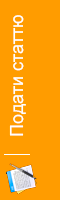#### Оніщенко В. О.

Населений пункт:
Київ, Україна

Кількість публікацій: 1

Summary: The paper presents the mathematical model of the process contact membrane distillation. The proposed simplified mathematical model of СMD , which takes into account the change in the temperature of the solution and the distillate channel along the membrane and the membrane module height . As well as describing the change in temperature of steam flow in the pores of the membrane. An algorithm for calculating mathematical model of contact membrane distillation.

Keywords: mathematical model, contact membrane distillation, heat transfer, mass transfer, distillate channel.

Галузь науки: Хімічні науки